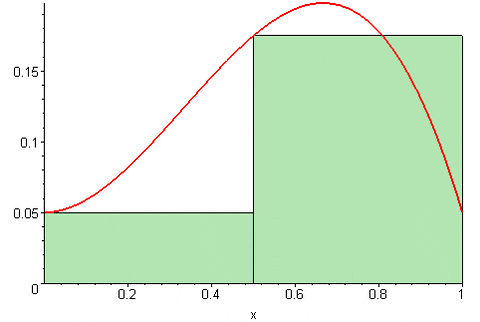# 106 ريض(Math106 (Integral Calculus

The definite integral, fundamental theorem of calculus, the indefinite integral, change of variable, numerical integration.  Differentiation and integration of inverse trigonometric functions. The logarithmic, exponential, hyperbolic and inverse hyperbolic functions. Techniques of integration:  by parts, trigonometric substitutions, partial fractions, miscellaneous substitutions. Indeterminate forms, improper integrals. Area, volume of revolution, work, arc length. Polar coordinates

ملحقات المادة الدراسية